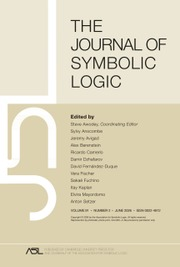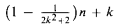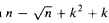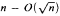Home
Hostname: page-component-79b67bcb76-c5xhk Total loading time: 0.247 Render date: 2021-05-14T21:13:31.474Z Has data issue: true Feature Flags: { "shouldUseShareProductTool": true, "shouldUseHypothesis": true, "isUnsiloEnabled": true, "metricsAbstractViews": false, "figures": false, "newCiteModal": false, "newCitedByModal": true, "newEcommerce": true }The Journal of Symbolic Logic

# Descriptive complexity of finite structures: Saving the quantifier rank

Published online by Cambridge University Press:  12 March 2014

Corresponding

## Abstract

We say that a first order formula Φ distinguishes a structure M over a vocabulary L from another structure M′ over the same vocabulary if Φ is true on M but false on M′. A formula Φ defines an L-structure M if Φ distinguishes M from any other non-isomorphic L-structure M′. A formula Φ identifies an n-element L-structure M if Φ distinguishes M from any other non-isomorphic n-element L-structure M′.

We prove that every n-element structure M is identifiable by a formula with quantifier rank less thanand at most one quantifier alternation, where k is the maximum relation arity of M. Moreover, if the automorphism group of M contains no transposition of two elements, the same result holds for definability rather than identification.

The Bernays-Schönfinkel class consists of prenex formulas in which the existential quantifiers all precede the universal quantifiers. We prove that every n-element structure M is identifiable by a formula in the Bernays-Schönfinkel class with less thanquantifiers. If in this class of identifying formulas we restrict the number of universal quantifiers to k, then less thanquantifiers suffice to identify M and. as long as we keep the number of universal quantifiers bounded by a constant, at totalquantifiers are necessary.

Type
Research Article
Information
The Journal of Symbolic Logic , June 2005 , pp. 419 - 450

## Access options

Get access to the full version of this content by using one of the access options below.

## References

 Börger, E., Grädel, E., and Gurevich, Y., The classical decision problem, Springer-Verlag, 1997.CrossRefGoogle Scholar
 Cai, J.-Y., Fürer, M., and Immerman, N., An optimal lower bound on the number of variables for graph identification, Combinatorica, vol. 12 (1992), pp. 389410.CrossRefGoogle Scholar
 Ebbinghaus, H.-D. and Flum, J., Finite model theory, 2nd rev. ed., Springer-Verlag, 1999.Google Scholar
 Ehrenfeucht, A., An application of games to the completeness problem for formalized theories, Fundamenta Mathematicae, vol. 49 (1961), pp. 129141.Google Scholar
 Fagin, R., Finite-model theory—a personal perspective, Theoretical Computer Science, vol. 116 (1993), pp. 331.CrossRefGoogle Scholar
 Fraïssé, R., Sur quelques classifications des systems de relations, Université d'Alger, Publications Scientifiques, Serie A, vol. 1 (1954), pp. 35182.Google Scholar
 Grohe, M., Fixed-point logics on planar graphs, Proceedings of the Annual Conference on Logic in Computer Science, 1998, pp. 615.Google Scholar
 Grohe, M., isomorphism testing for embeddable graphs through definability, Proceedings of the 32nd ACM Annual Symposium on Theory of Computing (STOC), 2000, pp. 6372.Google Scholar
 Immerman, N., Descriptive complexity, Springer-Verlag, 1999.CrossRefGoogle Scholar
 Immerman, N. and Kozen, D., Definability with bounded number of bound variables, Information and Computation, vol. 83 (1989), pp. 121139.CrossRefGoogle Scholar
 Immerman, N. and Lander, E., Describing graphs: a first-order approach to graph canonization, Complexity theory retrospective (Selman, A., editor), Springer-Verlag, 1990, pp. 5981.CrossRefGoogle Scholar
 Kim, J. H., Pikhurko, O., Spencer, J., and Verbitsky, O., How complex are random graphs in first order logic?, to appear in Random Structures and Algorithms, E-print http://arxiv.org/abs/math.C0/0401247, 2004.Google Scholar
 Pezzoli, E., Computational complexity of Ehrenfeucht-Fraïssé games on finite structures, Proceedings of the CSL '98 conference (Gottlob, G. and Seyr, K., editors). Lecture Notes in Computer Science, vol. 1584, Springer-Verlag, 1999, pp. 159170.Google Scholar
 Pikhurko, O., Spencer, J., and Verbitsky, O., Succinct definitions in first order graph theory, submitted, E-print http://arxiv.org/abs/math.C0/0401307, 2004.Google Scholar
 Pikhurko, O., Veith, H., and Verbitsky, O., First order definability of graphs: tight bounds on quantifier rank, submitted, E-print http://arxiv.org/abs/math.C0/0311041, 2003.Google Scholar
 Pikhurko, O. and Verbitsky, O., Descriptive complexity of finite structures: saving the quantifier rank, E-print http.-//arxiv.org/abs/math.L0/0305244, 2003.Google Scholar
 Spencer, J., The strange logic of random graphs, Springer-Verlag, 2001.CrossRefGoogle Scholar

# Send article to Kindle

Note you can select to send to either the @free.kindle.com or @kindle.com variations. ‘@free.kindle.com’ emails are free but can only be sent to your device when it is connected to wi-fi. ‘@kindle.com’ emails can be delivered even when you are not connected to wi-fi, but note that service fees apply.

Find out more about the Kindle Personal Document Service.

Descriptive complexity of finite structures: Saving the quantifier rank
Available formats
×

# Send article to Dropbox

To send this article to your Dropbox account, please select one or more formats and confirm that you agree to abide by our usage policies. If this is the first time you use this feature, you will be asked to authorise Cambridge Core to connect with your <service> account. Find out more about sending content to Dropbox.

Descriptive complexity of finite structures: Saving the quantifier rank
Available formats
×

# Send article to Google Drive

To send this article to your Google Drive account, please select one or more formats and confirm that you agree to abide by our usage policies. If this is the first time you use this feature, you will be asked to authorise Cambridge Core to connect with your <service> account. Find out more about sending content to Google Drive.

Descriptive complexity of finite structures: Saving the quantifier rank
Available formats
×
×